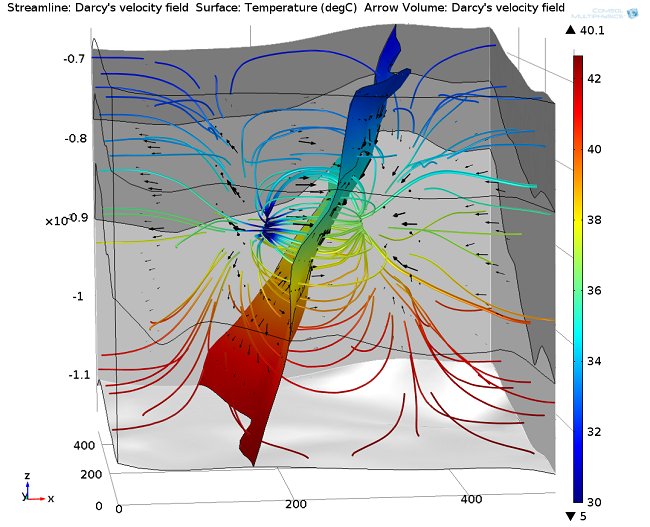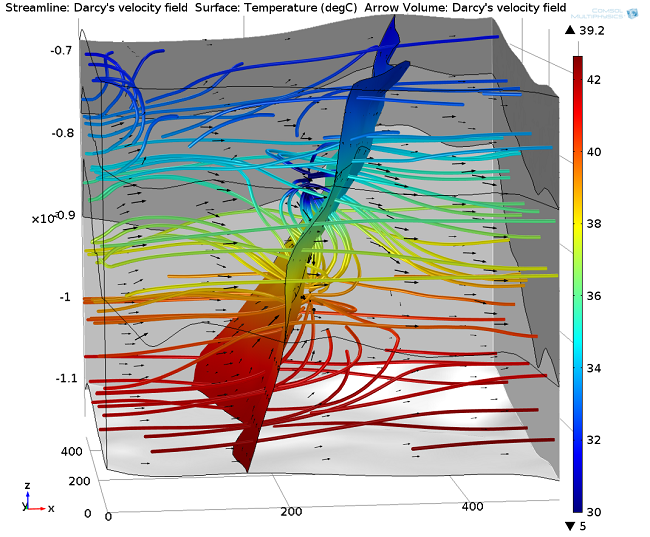# 传热与地下多孔介质流的耦合仿真

2014年 4月 24日

### 地下水换热系统中的方程

(1)

(\rho C_p)_{eq} \frac{\partial T}{\partial t} + \rho C_p {\bf u } \cdot \nabla T = \nabla \cdot (k_{eq} \nabla T ) + Q + Q_{geo}

(2)

(\rho C_p )_{eq} = \sum_{i} ( \theta_{pi}\rho_{pi}C_{p,pi})+(1-\sum_{i}\theta_{pi})\rho C_p

(3)

k_{eq}=\sum_{i} \theta_{pi} k_{pi} + ( 1-\sum_{i} \theta_{pi} ) \rho C_p

(4)

{\mathbf u} = -\frac{\kappa}{\mu} \nabla p

(5)

\frac{\partial}{\partial t} (\rho \epsilon_p) + \nabla \cdot ( \rho {\bf u} ) = Q_m

### 地下水换热应用的 COMSOL 模型：地热对井回灌### 延伸阅读

#### 评论 (4)

##### 留言##### chunhu zhao
2017-12-28##### Phillip Oberdorfer
2017-12-28

http://cn.comsol.com/model/geothermal-doublet-29751##### 春伟 周
2019-06-172021-02-23